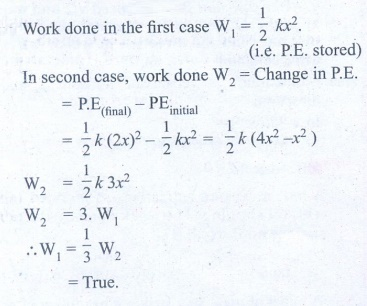Home | | Physics 11th std | Conceptual Questions Answers

## Chapter: 11th Physics : UNIT 4 : Work, Energy and Power

Physics : Work, Energy and Power : Book Back Exercise, Example Numerical Question with Answers, Solution : Conceptual Questions Answers

## Conceptual Questions

1. A spring which in initially in un-stretched condition, is first stretched by a length x and again by a further length x. The work done in the first case W1 is one third of the work done in second case W2. True or false?2. Which is conserved in inelastic collision? Total energy (or) Kinetic energy?

Total energy is always conserved.

But K.E. is not conserved.

3. Is there any net work done by external forces on a car moving with a constant speed along a straight road?

No.

If a car is moving at a constant speed, then external force will be zero.

Because a = [v - u] / tFor constant speed v = u, then a=0. (a-acceleration)

F = ma   .'. F = zero. i.e.. no external force.

W = F.S. = 0. So net work done is zero.

4. A car starts from rest and moves on a surface with uniform acceleration.

Draw  the  graph  of  kinetic  energy versus displacement. What information you can get from that graph?5. A charged particle moves towards another charged particle. Under what conditions the total momentum and the total energy of the system conserved?